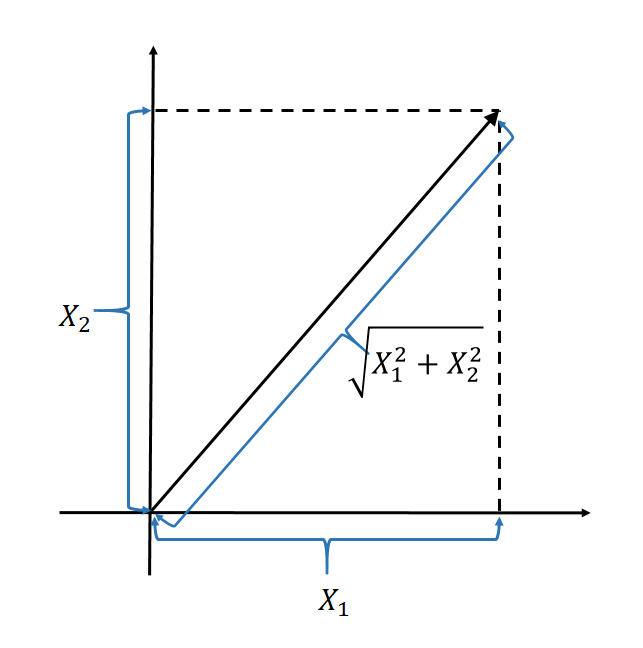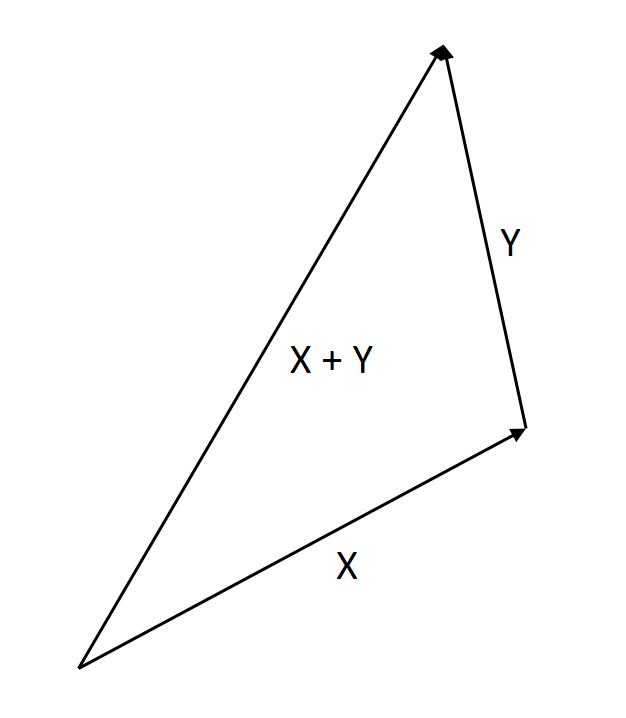26
Nov 16

## Properties of correlation

### Correlation coefficient: the last block of statistical foundation

Correlation has already been mentioned in

Statistical measures and their geometric roots

Properties of standard deviation

The pearls of AP Statistics 35

Properties of covariance

The pearls of AP Statistics 33

### The hierarchy of definitions

Suppose random variables$X,Y$ are not constant. Then their standard deviations are not zero and we can define their correlation as in Chart 1.Chart 1. Correlation definition

### Properties of correlation

Property 1. Range of the correlation coefficient: for any$X,Y$ one has$- 1 \le \rho (X,Y) \le 1$.
This follows from the Cauchy-Schwarz inequality, as explained here.

Recall from this post that correlation is cosine of the angle between$X-EX$ and$Y-EY$.
Property 2. Interpretation of extreme cases. (Part 1) If$\rho (X,Y) = 1$, then$Y = aX + b$ with$a > 0.$

(Part 2) If$\rho (X,Y) = - 1$, then$Y = aX + b$ with$a < 0$.

Proof. (Part 1)$\rho (X,Y) = 1$ implies
(1)$Cov (X,Y) = \sigma (X)\sigma (Y)$
which, in turn, implies that$Y$ is a linear function of$X$:$Y = aX + b$ (this is the second part of the Cauchy-Schwarz inequality). Further, we can establish the sign of the number$a$. By the properties of variance and covariance$Cov(X,Y)=Cov(X,aX+b)=aCov(X,X)+Cov(X,b)=aVar(X)$,$\sigma (Y)=\sigma(aX + b)=\sigma (aX)=|a|\sigma (X)$.
Plugging this in Eq. (1) we get$aVar(X) = |a|\sigma^2(X)$ and see that$a$ is positive.

The proof of Part 2 is left as an exercise.

Property 3. Suppose we want to measure correlation between weight$W$ and height$H$ of people. The measurements are either in kilos and centimeters${W_k},{H_c}$ or in pounds and feet${W_p},{H_f}$. The correlation coefficient is unit-free in the sense that it does not depend on the units used:$\rho (W_k,H_c)=\rho (W_p,H_f)$. Mathematically speaking, correlation is homogeneous of degree$0$ in both arguments.
Proof. One measurement is proportional to another,$W_k=aW_p,\ H_c=bH_f$ with some positive constants$a,b$. By homogeneity$\rho (W_k,H_c)=\frac{Cov(W_k,H_c)}{\sigma(W_k)\sigma(H_c)}=\frac{Cov(aW_p,bH_f)}{\sigma(aW_p)\sigma(bH_f)}=\frac{abCov(W_p,H_f)}{ab\sigma(W_p)\sigma (H_f)}=\rho (W_p,H_f).$

13
Nov 16

## Statistical measures and their geometric roots

Variance, covariancestandard deviation and correlation: their definitions and properties are deeply rooted in the Euclidean geometry.

## Here is the why: analogy with Euclidean geometryEuclid axiomatically described the space we live in. What we have known about the geometry of this space since the ancient times has never failed us. Therefore, statistical definitions based on the Euclidean geometry are sure to work.

#### 1. Analogy between scalar product and covariance

Geometry. See Table 2 here for operations with vectors. The scalar product of two vectors$X=(X_1,...,X_n),\ Y=(Y_1,...,Y_n)$ is defined by$(X,Y)=\sum X_iY_i.$

Statistical analog: Covariance of two random variables is defined by$Cov(X,Y)=E(X-\bar{X})(Y-\bar{Y}).$

Both the scalar product and covariance are linear in one argument when the other argument is fixed.

#### 2. Analogy between orthogonality and uncorrelatedness

Geometry. Two vectors$X,Y$ are called orthogonal (or perpendicular) if

(1)$(X,Y)=\sum X_iY_i=0.$

Exercise. How do you draw on the plane the vectors$X=(1,0),\ Y=(0,1)$? Check that they are orthogonal.

Statistical analog: Two random variables are called uncorrelated if$Cov(X,Y)=0$.

#### 3. Measuring lengthsFigure 1. Length of a vector

Geometry: the length of a vector$X=(X_1,...,X_n)$ is$\sqrt{\sum X_i^2}$, see Figure 1.

Statistical analog: the standard deviation of a random variable$X$ is$\sigma(X)=\sqrt{Var(X)}=\sqrt{E(X-\bar{X})^2}.$

This explains the square root in the definition of the standard deviation.

#### 4. Cauchy-Schwarz inequality

Geometry$|(X,Y)|\le\sqrt{\sum X_i^2}\sqrt{\sum Y_i^2}$.

Statistical analog$|Cov(X,Y)|\le\sigma(X)\sigma(Y)$. See the proof here. The proof of its geometric counterpart is similar.

#### 5. Triangle inequalityFigure 2. Triangle inequality

Geometry$\sqrt{\sum (X_i+Y_i)^2}\le\sqrt{\sum X_i^2}+\sqrt{\sum X_i^2}$, see Figure 2 where the length of X+Y does not exceed the sum of lengths of X and Y.

Statistical analog: using the Cauchy-Schwarz inequality we have$\sigma(X+Y)=\sqrt{Var(X+Y)}$$=\sqrt{Var(X)+2Cov(X,Y)+Var(Y)}$$\le\sqrt{\sigma^2(X)+2\sigma(X)\sigma(Y)+\sigma^2(Y)}$$=\sigma(X)+\sigma(Y).$

#### 4. The Pythagorean theorem

Geometry: In a right triangle, the squared hypotenuse is equal to the sum of the squares of the two legs. The illustration is similar to Figure 2, except that the angle between X and Y should be right.

Proof. Taking two orthogonal vectors$X,Y$ as legs, we have

Squared hypotenuse =$\sum(X_i+Y_i)^2$

(squaring out and using orthogonality (1))$=\sum X_i^2+2\sum X_iY_i+\sum Y_i^2=\sum X_i^2+\sum Y_i^2$ = Sum of squared legs

Statistical analog: If two random variables are uncorrelated, then variance of their sum is a sum of variances$Var(X+Y)=Var(X)+Var(Y).$

#### 5. The most important analogy: measuring angles

Geometry: the cosine of the angle between two vectors$X,Y$ is defined by

Cosine between X,Y =$\frac{\sum X_iY_i}{\sqrt{\sum X_i^2\sum Y_i^2}}.$

Statistical analog: the correlation coefficient between two random variables is defined by$\rho(X,Y)=\frac{Cov(X,Y)}{\sqrt{Var(X)Var(Y)}}=\frac{Cov(X,Y)}{\sigma(X)\sigma(Y)}.$

This intuitively explains why the correlation coefficient takes values between -1 and +1.

Remark. My colleague Alisher Aldashev noticed that the correlation coefficient is the cosine of the angle between the deviations$X-EX$ and$Y-EY$ and not between$X,Y$ themselves.